# AP Physics C: Mechanics : Understanding Accelerations

## Example Questions

### Example Question #11 : Mechanics Exam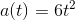A car undergoes acceleration according to the given function. What distance has the car traveled after three seconds?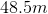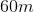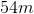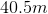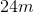Explanation:

We can determine the velocity by taking the second integral of acceleration for the time interval of 0s to 3s.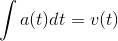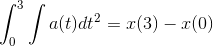Solve for the first integral.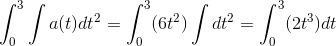Solve for the second integral, using the time interval.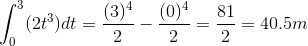### Example Question #12 : Mechanics ExamA car undergoes acceleration according to the given function. How fast is the car moving after four seconds?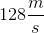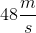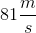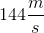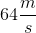Explanation:

We can find the car's velocity by taking the integral of the acceleration function during the given time interval.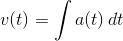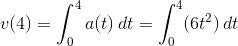Solve the integral for the time interval of 0s to 4s. This will give us the final velocity.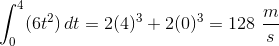### Example Question #13 : Mechanics ExamA car undergoes acceleration according to the given function. If the threshold for serious injury or fatality for a human undergoing horizontal acceleration is 60 gees (1 gee = 10 meters per second per second), how long would a human be able to withstand riding in this car?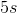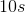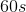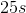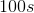Explanation:

Calculate the maximum acceration in meters per second.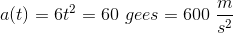Solve for the time.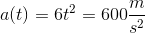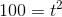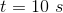### Example Question #14 : Mechanics Exam

A ball travels with a velocity as described by the function below: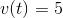What is the ball's acceleration?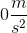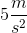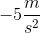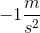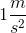Explanation:

Acceleration is equal to the derivative of the velocity function.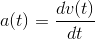Since the velocity is constant, the derivative will be equal to zero.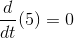The acceleration is equal to zero.

### All AP Physics C: Mechanics Resources